# Python++

linux.conf.au 2019

Jan Groth

• DevOps Engineer at Versent* in Sydney**
• Developer Background

(*) We are hiring
(**) In Sydney and Melbourne

## This talk

• Here are 10 Python features - now start using them!
• Things that I find useful when writing code in Python
• Don't take notes

## What year is this?

• The Dark Knight is the most popular movie
• Lehman Brothers file for bankruptcy
• Spotify launches in Sweden
• Lady Gaga has number-one singles with Just Dance and Poker Face

# 2008

Python 3.0 was released on December 3, 2008
It was designed to rectify fundamental design flaws in the language—the changes required could not be implemented while retaining full backwards compatibility with the 2.x series, which necessitated a new major version number

Source: Wikipedia

Python 2

or

Python 3?

Best reason to stop using Python 2.7 now:

Python versions and project dependencies

One Python environment per project

! Don't use the system environment

## Tip #1

• Use Python 3
• Use a tool to manage environments
• venv
• pipenv

More conventions - less thinking ...

```                    ```
CONSTANTS_IN_UPPERCASE = 123

variables_are_lowercase = 'OH YES!'

def methods_are_lowercase_too():
pass

def all_use_snake_case():
pass
```
```

File names...

```                    ```
lambda.py
nexus.py
```
```
```                    ```
rewards-calculation-lambda.py
query-nexus-for-latest-snapshot-version.py
```
```

Complex code ...

```                    ```
# scale out if less than 3 instances
if len(current_instances) < 3:
```
```
```                    ```
if should_scale_out(current_instances):
```
```

Complex code ...

```                    ```
# scale out if less than 3 instances
if len(current_instances) < 3:
```
```
```                    ```
if should_scale_out(current_instances):
```
```

## Tip #2

Use naming conventions

Make an effort to find good names

## Writing beautiful code

There is beauty in simplicity.

Using the language construct that fits best

```                        ```
counter = 0
while counter < 5:
print(counter)
counter += 1
```
```
```                        ```
for i in range(5):
print(i)
```
```
There is often a pythonic way

Loop vs List Comprehension

```                        ```
symbols = '\$¢£¥€¤'
codes = []
for symbol in symbols:
codes.append(ord(symbol))

>>> [36, 162, 163, 165, 8364, 164]
```
```
```                        ```
symbols = '\$¢£¥€¤'
codes = [ord(symbol) for symbol in symbols]

>>> [36, 162, 163, 165, 8364, 164]
```
```

Loop vs List Comprehension

```                        ```
symbols = '\$¢£¥€¤'
codes = []
for symbol in symbols:
codes.append(ord(symbol))

>>> [36, 162, 163, 165, 8364, 164]
```
```
```                        ```
symbols = '\$¢£¥€¤'
codes = [ord(symbol) for symbol in symbols]

>>> [36, 162, 163, 165, 8364, 164]
```
```

Commenting every single line is essential ...

..if you are writing in Assembler.

```                    ```
flash2ram:
lpm                 ;get constant
st      Y+,r0       ;store in SRAM and increment Y-pointer
dec     flashsize
brne    flash2ram   ;if not end of table, loop more
ret
```
```

In Python not so much.

```                    ```
# Randomise Salt
salt = os.urandom(6)
# Convert String to Byte Array
res = arg.encode()
# Hash through 10000 times.
for lp in range(100000):
# Always initialize hashlib, because [...]
m = hashlib.sha256()
# Swap between the two inputs into the digest based on loop number
m.update(res) if lp % 2 else m.update(salt)
m.update(salt) if lp % 2 else m.update(res)
# Finish the digest
res = m.digest()
```
```

In Python not so much.

```                    ```
# Randomise Salt
salt = os.urandom(6)
# Convert String to Byte Array
res = arg.encode()
# Hash through 10000 times.
for lp in range(100000):
# Always initialize hashlib, because [...]
m = hashlib.sha256()
# Swap between the two inputs into the digest based on loop number
m.update(res) if lp % 2 else m.update(salt)
m.update(salt) if lp % 2 else m.update(res)
# Finish the digest
res = m.digest()
```
```

## Tip #3

#### Less is more

Write code that is both functional and simple

Use Python idioms where you can

Comment only what you cannot say in code

## Classes

A basic calculator

Calculations on top of a base number

```                    ```
base = 10

def multiply(number):
return base * number

return base + number

print(multiply(5))  # ...50
```
```

Time passes ...

... new requirements are coming in:

Calculations on top of a second number

```                    ```
base = 10

def multiply(number):
return base * number

return base + number

print(multiply(5))  # ...50

.
```
```

Calculations on top of a second number

```                    ```
base = 10

def multiply(number):
return base * number

return base + number

print(multiply(5))  # ...50

base = 6
print(multiply(5))  # ...30
```
```

Problem: Separation of state...

```                    ```
base = 10

def multiply(number):
return base * number

return base + number

print(multiply(5))  # ...50

base = 6
print(multiply(5))  # ...30
```
```

...and behaviour

```                    ```
base = 10

def multiply(number):
return base * number

return base + number

print(multiply(5))  # ...50

base = 6
print(multiply(5))  # ...30
```
```

...make the code much harder to understand

```                    ```
base = 10

def multiply(number):
return base * number

return base + number

print(multiply(5))  # ...50

base = 6
print(multiply(5))  # ...30
```
```

#### Using classes to redesign the calculator

• A class is a blueprint for objects
• An object encapsulates data as well as methods

API Design first ...

```                    ```
calculator = Calculator(base=10)
print(calculator.multiply_by(5))
```
```

API Design first ...

```                    ```
calculator = Calculator(base=10)
print(calculator.multiply_by(5))
```
```

Implementation

```                    ```
class Calculator:
def __init__(self, base):
self.calc_base = base

def multiply_by(self, number):
return self.calc_base * number

return self.calc_base + number
```
```

Implementation

```                    ```
class Calculator:
def __init__(self, base):
self.calc_base = base

def multiply_by(self, number):
return self.calc_base * number

return self.calc_base + number
```
```

Implementation

```                    ```
class Calculator:
def __init__(self, base):
self.calc_base = base

def multiply_by(self, number):
return self.calc_base * number

return self.calc_base + number
```
```

Implementation

```                    ```
class Calculator:
def __init__(self, base):
self.calc_base = base

def multiply_by(self, number):
return self.calc_base * number

return self.calc_base + number
```
```

Different calculators are now separate objects ...

```                    ```
base_ten_calc = Calculator(base=10)
print(base_ten_calc.multiply_by(5)) # ...50

base_six_calc = Calculator(base=6)
print(base_six_calc.multiply_by(5)) # ...30
```
```

Different calculators are now separate objects ...

```                    ```
base_ten_calc = Calculator(base=10)
print(base_ten_calc.multiply_by(5)) # ...50

base_six_calc = Calculator(base=6)
print(base_six_calc.multiply_by(5)) # ...30
```
```

A real-world example -
Syncing a file between SFTP and S3

```                    ```
s2s_sync = Sftp2S3(server_name='a.b.c', bucket_name='my-bucket')

s2s_sync.sync_file('foo.txt')
s2s_sync.sync_file('baz/bar.txt')
```
```

A real-world example -
Syncing a file between SFTP and S3

```                    ```
s2s_sync = Sftp2S3(server_name='a.b.c', bucket_name='my-bucket')

s2s_sync.sync_file('foo.txt')
s2s_sync.sync_file('baz/bar.txt')
```
```

A real-world example -
Syncing a file between SFTP and S3

```                    ```
s2s_sync = Sftp2S3(server_name='a.b.c', bucket_name='my-bucket')

s2s_sync.sync_file('foo.txt')
s2s_sync.sync_file('baz/bar.txt')
```
```

Implementation Design

```                    ```
class Sftp2S3:
def __init__(self, server_name, bucket_name):
self.sftp_server = self._init_sftp(server_name)
self.bucket = boto3.resource('s3').Bucket(bucket_name)

def _init_sftp(self, server_name): pass
def _needs_sync(self, file_name): pass
def _copy_from_sftp(self, file_name): pass

def sync_file(self, file_name):
if (self._needs_sync(file_name)):
self._copy_from_sftp(file_name)
```
```

Implementation Design

```                    ```
class Sftp2S3:
def __init__(self, server_name, bucket_name):
self.sftp_server = self._init_sftp(server_name)
self.bucket = boto3.resource('s3').Bucket(bucket_name)

def _init_sftp(self, server_name): pass
def _needs_sync(self, file_name): pass
def _copy_from_sftp(self, file_name): pass

def sync_file(self, file_name):
if (self._needs_sync(file_name)):
self._copy_from_sftp(file_name)
```
```

Implementation Design

```                    ```
class Sftp2S3:
def __init__(self, server_name, bucket_name):
self.sftp_server = self._init_sftp(server_name)
self.bucket = boto3.resource('s3').Bucket(bucket_name)

def _init_sftp(self, server_name): pass
def _needs_sync(self, file_name): pass
def _copy_from_sftp(self, file_name): pass

def sync_file(self, file_name):
if (self._needs_sync(file_name)):
self._copy_from_sftp(file_name)
```
```

#### This is just a sneak peek into classes

• Re-use code elsewhere
• Utilize inheritance to implement your own generators, collections ...
• And ...

Unit Testing

```                    ```
class TestCalculator(TestCase):

def test_multiply_with_zero_is_zero(self):
# setup
calc = Calculator(29)

# exercise
result = calc.multiply_by(0)

# verify
self.assertEqual(0, result)
```
```

## Tip #4

Encapsulate behaviour as well as state

Use classes - The door to a whole new world

## The right tool for the job

What does this code print?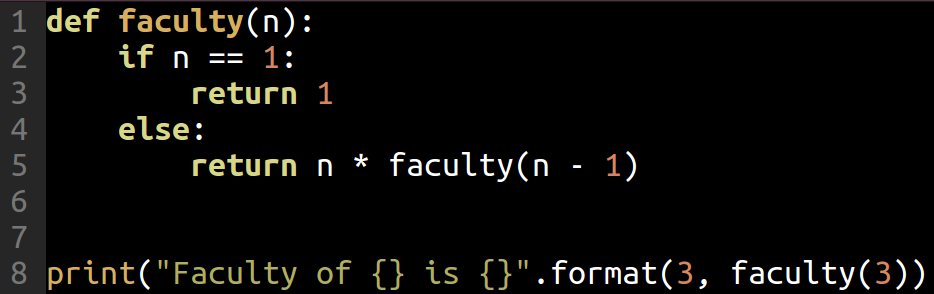... not much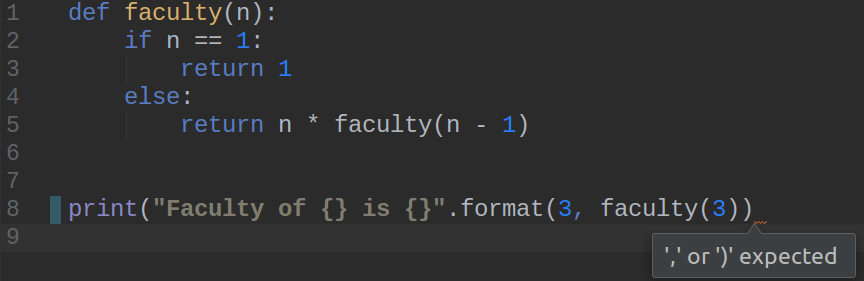Can vim emacs sublime a text editor do this?

Remove unused imports ...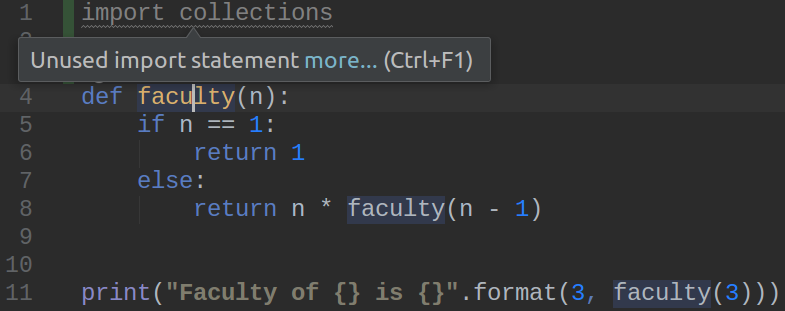Reformat code according to Python standards ...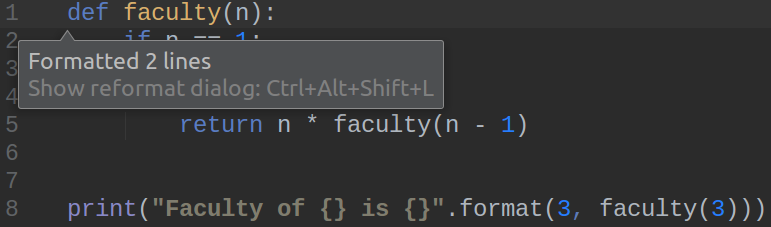Throw in a breakpoint ...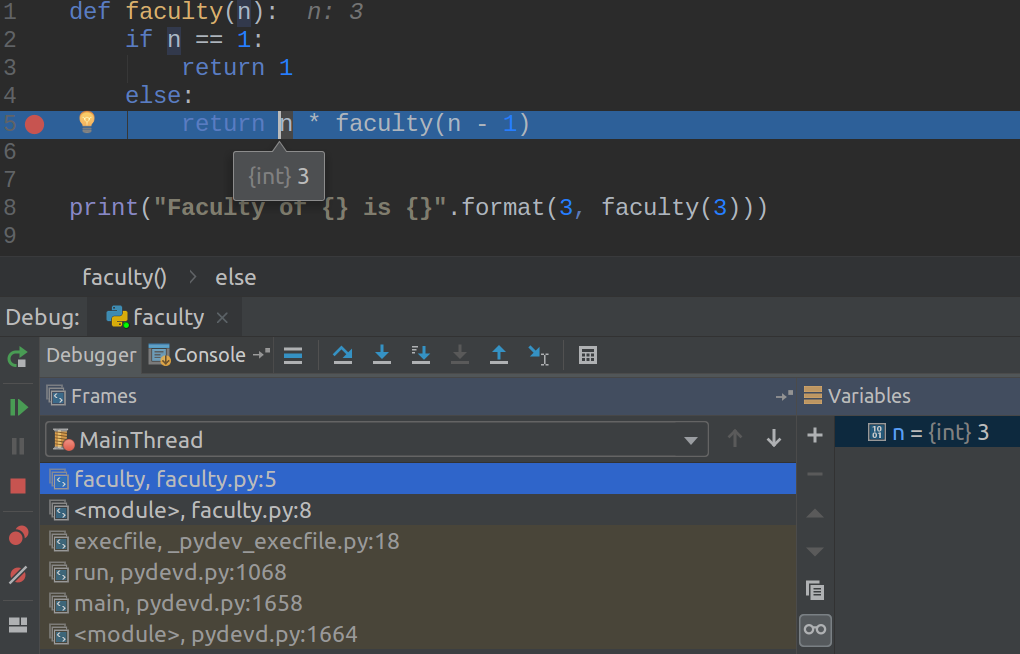Change a method name across the whole project ...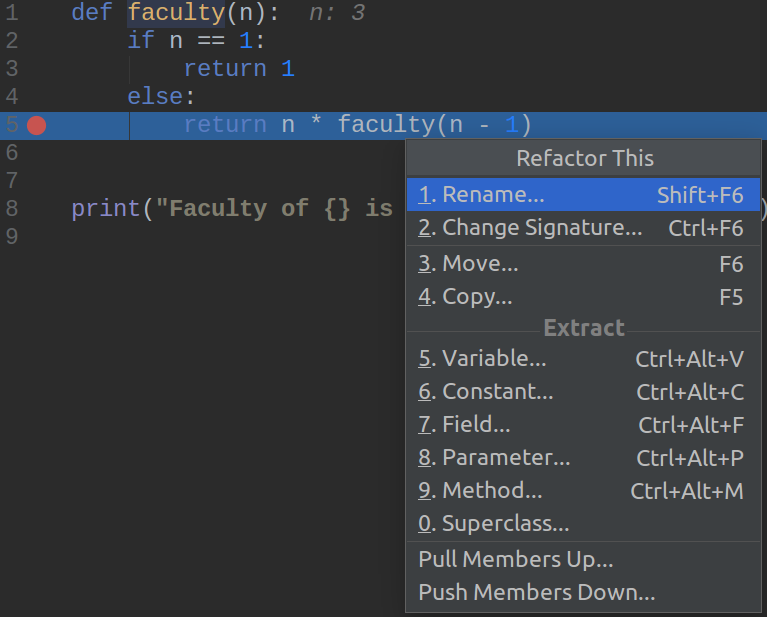## Tip #5

Python is a high-level programming language.

Use an IDE.

Just because you can.

## The End ☺

I hope you find it useful
Or ...

Tim Peters, Python core developer:

Here's the plan:
When someone uses a feature you don’t understand, simply shoot them. This is easier than learning something new, and before too long the only living coders will be writing in an easily understood, tiny subset of Python 0.9.6 ;-)

The talk
jangroth.github.io/linuxconf2019